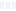Analytics

You can quickly run various types of analytics such as statistical tests, statistical and machine learning models, clustering, time series forecasting, survival analysis, etc. with a matter of a few clicks and discover hidden trends and patterns with preconfigured rich visualizations.

Statistical Test
Various types of Statistical tests (t-test, ANOVA, Wilcoxon test, Kruskal-Wallis test, Chi-Square test, etc.) are supported in Exploratory.

You can find deeper insights with a series of useful metrics such as effect size, power, P-value, confidence interval, etc. along with a set of preconfigured visualizations.Statistical Test with Multiple Groups
You can run the test with multiple groups together to make your analysis deeper.Power Analysis
Calculate the required sample size with the power analysis feature.Prediction Models for Data Analysis
You can quickly build various types of Statistical, Machine Learning, and Survival prediction models to understand the relationship between the variables, test the models, and predict the future.
Statistical Learning
• Linear Regression
• Logistic Regression
• GLM
• Cox Regression
Machine Learning
• Decision Tree
• Random Forest
• XGBoost
• Random Survival Forest

A Grammar of Prediction Model
Regardless of whether Statistical or Machine Learning models, Exploratory provides you a consistent experience with a set of preconfigured rich visualizations to help you understand the models intuitively and discover hidden trends and patterns quickly.

Variable Importance
Find out which variables are more useful to predict a variable of your interest.Prediction by Variable
Find out how a change in each variable impacts the prediction.Coefficients
Find the parameters for statistical models such as coefficients, odds ratios, hazard ratios along with confidence intervals.Multiple Models for Multiple Groups
Often, a single model doesn’t fit everybody. You can split data into multiple groups and fit a model to each group. Some groups might fit better than the others and you might find different patterns among the groups.Diagnostics - Multicollinearity
Use various diagnostic tools to build better prediction models.K-Means Clustering
Find the similarities among the groups and understand why they are similar or different from one another with a set of preconfigured rich visualizations.Decision Tree
Find the hidden rules that are embedded inside the data with Decision Tree.Survival Analysis
Sometimes, it’s not about if, it’s about when it happens. Use a family of survival analysis algorithms like Cox Regression, Random Survival Forest, and Kaplan-Meier to analyze how and when a given event happens.Use Market Basket Analysis algorithm to find out what are the things purchased or happen together more often than the others and how they are related.Time Series Forecasting
Not only forecast the future based on the past but also gain deeper insights on what other variables might be associated with the forecast and how seasonality plays a role in your data with cutting edge algorithms like Prophet.Time Series Anomaly Detection
Automatically detect anomaly in your time series data with Anomaly Detection algorithm.Principal Component Analysis
Find which variables are correlated to one another and how they are correlated with a set of rich visualizations.